Quantity: 0

Total: 0,00

0

# Cylindrical solids

### Cylindrical solids

This animation demonstrates various types of cylindrical solids as well as their lateral surfaces.

Mathematics

Keywords

cylindrical solid, right circular cylinder, oblique circular cylinder, right prism, right rectangular prism, lateral surface, base circle, rectangle, solid geometry, mathematics

Related items

### Scenes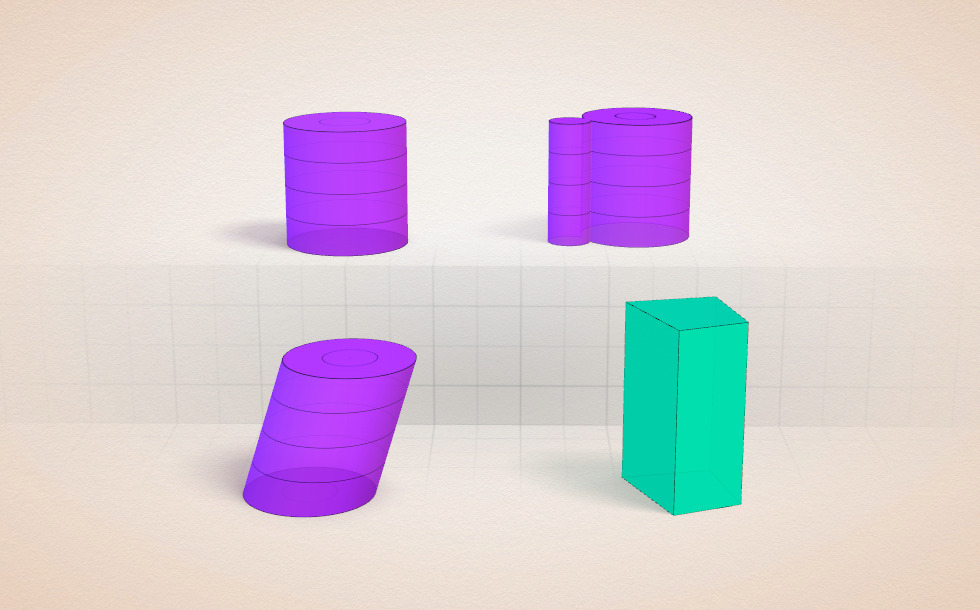### Cylindrical solids

• right circular cylinder
• oblique circular cylinder
• cylindrical solid
• right rectangular prism

There are two kinds of cylindrical solids: right cylinders and oblique cylinders. A cylinder with all generatrices perpendicular to the base is a right cylinder. A cylinder with generatrices not perpendicular to the base is an oblique cylinder. A cylinder with a polygonal base is a prism.

If the length of the generatrix and the height are equal, the cylinder is a right cylinder:

g=h

The lateral surface of right cylinders is rectangular. The lateral surface is a rectangle; its two sides are equal to the height of the cylinder and the circumference of the base, respectively.

If the generatrix is longer than the height, the cylinder is an oblique cylinder:

g>h

Surface: The surface area of a cylinder is the sum of the areas of the two bases and the area of the lateral surface:In the case of the right circular cylinder:Volume: To calculate the volume of the cylinder we need to multiply the surface area of the base by the height of the cylinder:In the case of right circular cylinders, the volume is: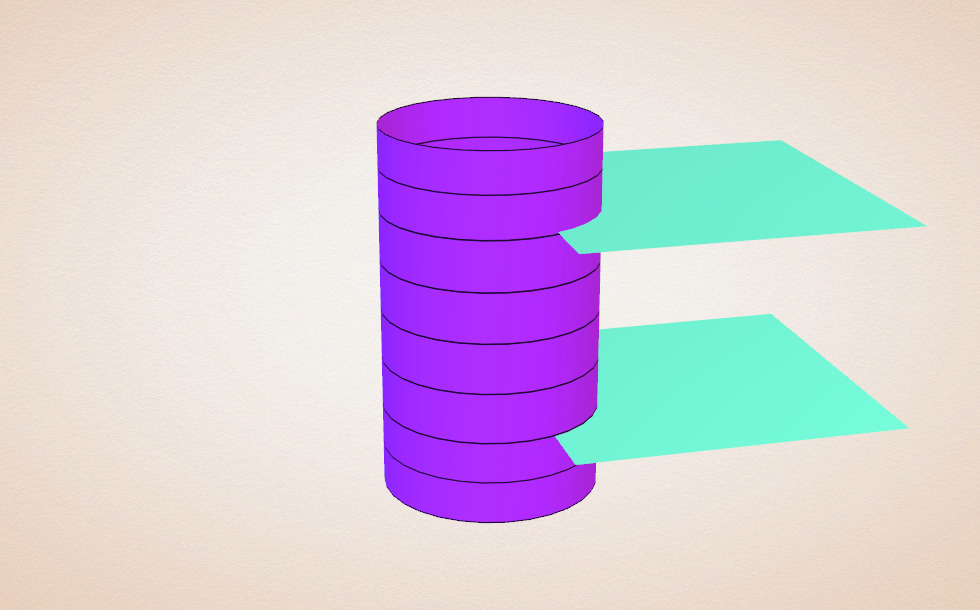## Derivation of cylinders

Let´s consider a geometric shape. Let´s draw a perpendicular line from a point on the closed curve, bordering the geometric shape, to the plane of the geometric shape. Let´s rotate this line parallel to itself around the border of the curve. Let´s intersect the surface formed by rotating the line with a plane parallel to the base. The solid defined by the cross section, the surface created as a result of rotating the line, i.e. the surface between the two cross sections is called a right cylinder. The cross section defines a geometric shape identical with the original one. The two coinciding geometric shapes are called the bases of the cylinder, the segment created by the line is called the generatrix, while the surface defined by the generatrices is called the lateral surface of the cylinder.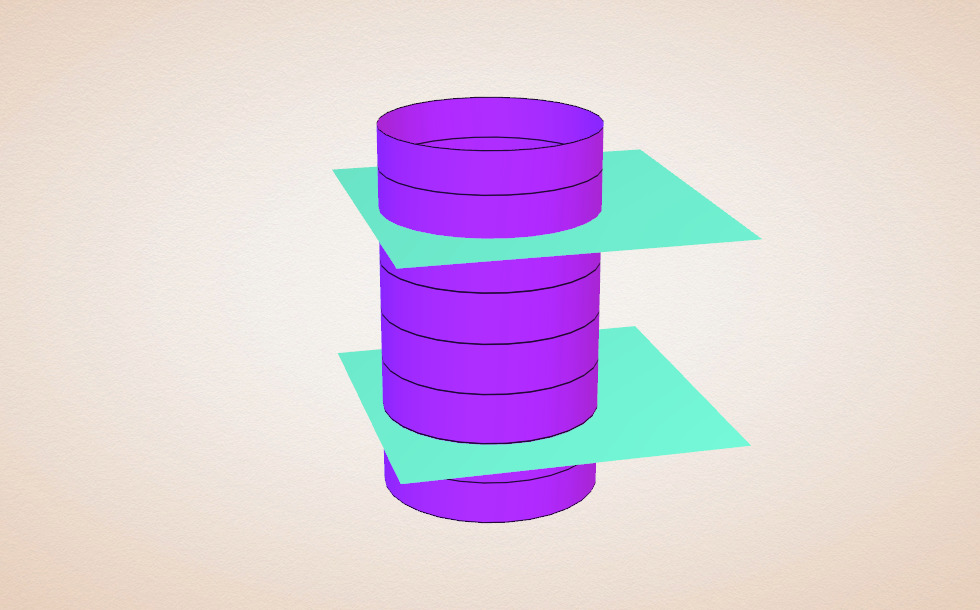### Right circularcylinder

• g=h
• base
• lateral surface

## Right circular cylinder

If the length of the generatrix and of the height are equal, the cylinder is a right cylinder:

g=h

The lateral surface of right cylinders is rectangular. You can easily check this by pressing the ˝Net˝ button. The lateral surface is a rectangle; its two sides are equal to the height of the cylinder and the circumference of the base, respectively.

If the bottom base of a right cylinder is circular, the cylinder is a right circular cylinder.

Surface: The surface area of a cylinder is the sum of the surface area of the two bases and the area of the lateral surface:Volume: To calculate the volume of a cylinder we need to multiply the area of the base by the height of the cylinder: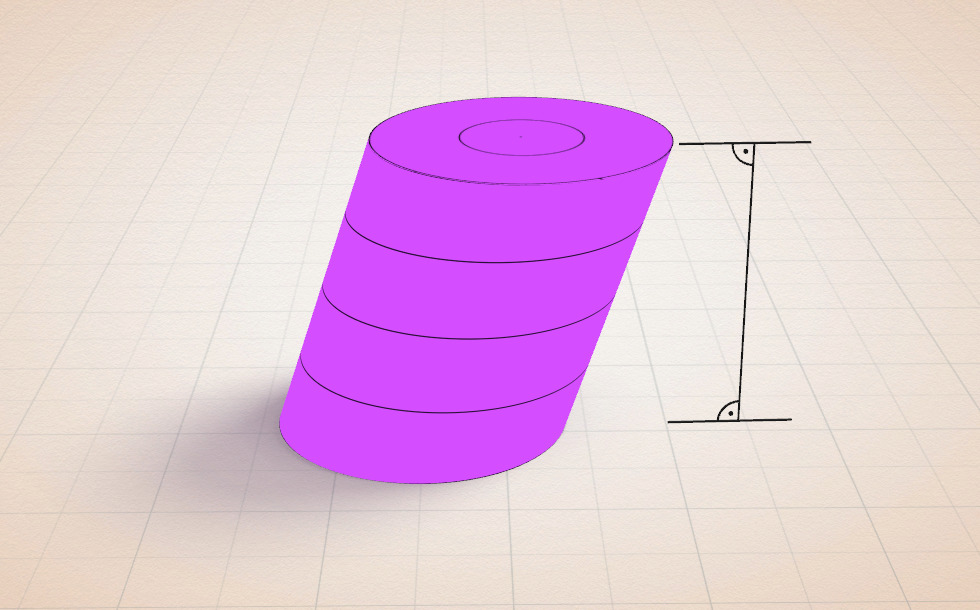### Oblique circularcylinder

• h
• base
• lateral surface

## Oblique circular cylinder

If the generatrix is longer than the height, the cylinder is an oblique cylinder:

g>h

Surface: The surface area of a cylinder is the sum of the surface area of the two bases and the area of the lateral surface:Volume: To calculate the volume of the cylinders we need to multiply the area of the base by the height of the cylinder: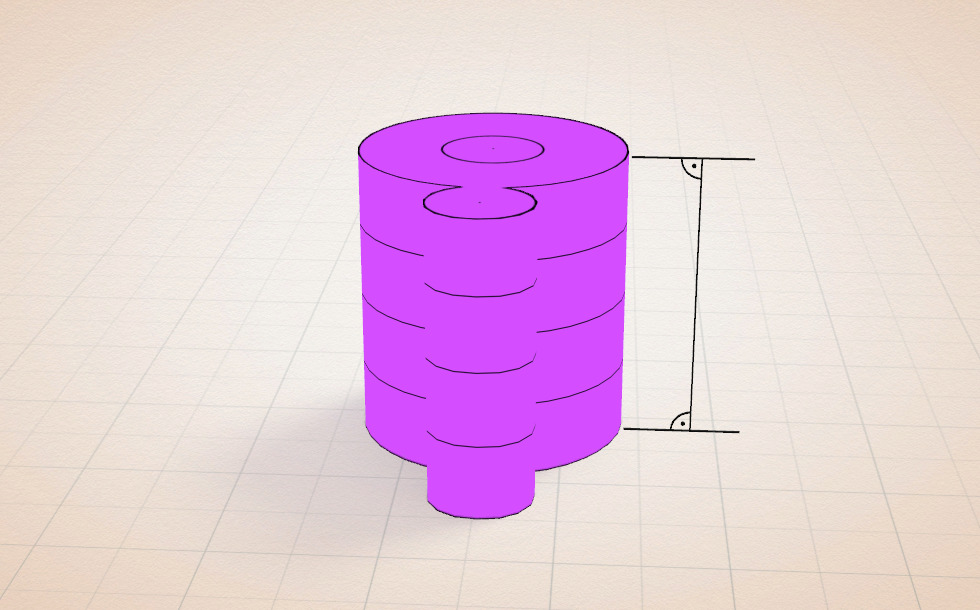### Cylindrical solid

• g=h
• base
• lateral surface

## Cylindrical solids

If the length of the generatrix and of the height are equal, the cylinder is a right cylinder:

g=h

The lateral surface of right cylinders is rectangular. You can easily check this by pressing the ˝Net˝ button. The lateral surface is a rectangle; its two sides are equal to the height of the cylinder and the circumference of the base, respectively.

If the generatrix is longer than the height, the cylinder is an oblique cylinder:

g>h

Surface: The surface area of a cylinder is the sum of the surface area of the two bases and the area of the lateral surface:Volume: To calculate the volume of the cylinder we need to multiply the area of the base by the height of the cylinder: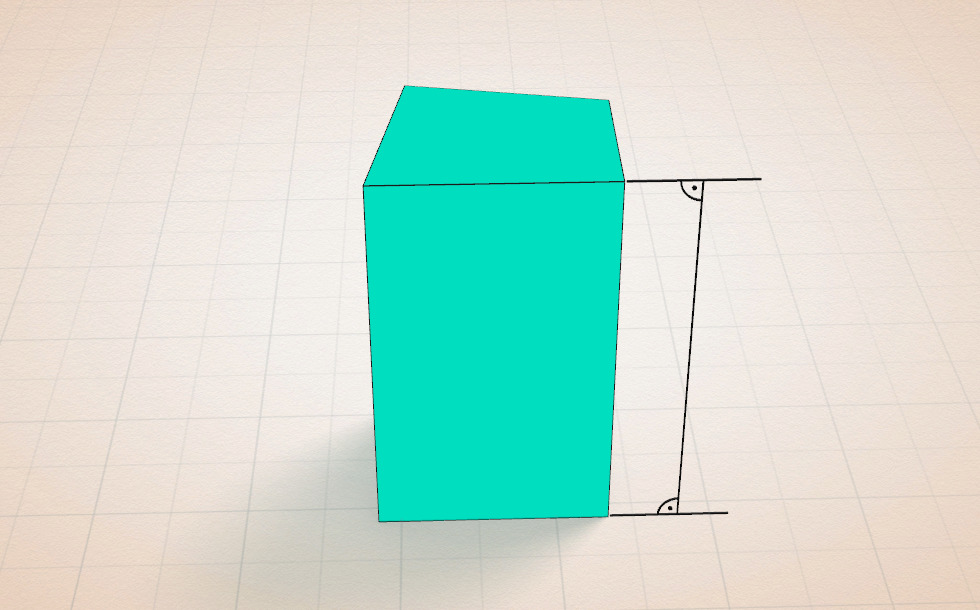• g=h

## Prism

Prisms are polygonal cylinders.
The lateral surfaces of right prisms, i. e. the sides, are rectangular.
The lateral surfaces of oblique prisms are parallelepipedic.

Surface area is the sum of the areas of the polygons that cover it. In other words the surface area is the sum of the areas of bases and sides:If we compare two prisms, a right and an oblique, with congruent polygon bases and heights, the surface area of the oblique prism is larger.
The volume is given by the area of a base times the height:If we compare two prisms, a right and an oblique, with congruent polygon bases and heights, the volumes of the two prisms are identical.

### Related items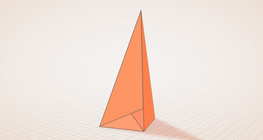#### Császár polyhedron

The Császár polyhedron is a nonconvex polyhedron with 14 triangular faces.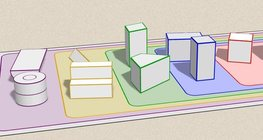#### Grouping of solids

This animation demonstrates various groups of solids through examples.#### Grouping of solids 1

This animation demonstrates various groups of solids through examples.#### Grouping of solids 2

This animation demonstrates various groups of solids through examples.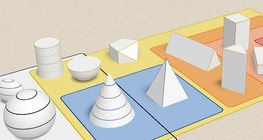#### Grouping of solids 3

This animation demonstrates various groups of solids through examples.#### Grouping of solids 4

This animation demonstrates various groups of solids through examples.#### Perimeter, area, surface area and volume

This animation presents the formulas to calculate the perimeter and area of shapes as well as the surface area and volume of solids.#### Platonic solids

This animation demonstrates the five regular three-dimensional (or Platonic) solids, the best known of which is the cube.#### Szilassi polyhedron

This special concave polyhedron was named after a Hungarian mathematician.#### Solids of revolution

Rotating a geometric shape around a line within its geometric plane as an axis results in a solid of revolution.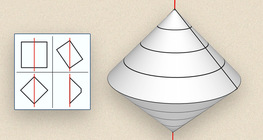#### Solids of revolution (exercise)

An exercise about the generation of solids of revolution.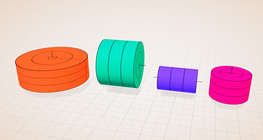#### Solids of revolution (rectangle)

Rotating a rectangle around its axes of symmetry or around its sides results in solids of revolution.#### Sphere

A sphere is the set of points which are all within the same distance from a given point in space.#### Conic solids

This animation demonstrates various types of cones and pyramids.